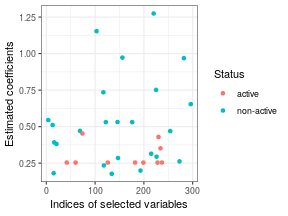# Introduction

This package provides functions for implementing the variable selection approach in high-dimensional linear models called WLasso described in . This method is designed for taking into account the correlations that may exist between the predictors (columns of the design matrix). It consists in rewriting the initial high-dimensional linear model to remove the correlation existing between the predictors and in applying the generalized Lasso criterion. We refer the reader to the paper for further details.

Let the response variable $$\boldsymbol{y}$$ satisfy the following linear model: \begin{equation}\label{eq:mod_lin} \boldsymbol{y}=\boldsymbol{X}\boldsymbol{\beta}+\boldsymbol{\epsilon}, \end{equation} where $$\boldsymbol{X}$$ is the design matrix having $$n$$ rows and $$p$$ columns, $$\boldsymbol{\beta}$$ is a vector of coefficients of size $$p$$ and $$\boldsymbol{\epsilon}$$ is the error term. The rows of $$\boldsymbol{X}$$ are assumed to be the realizations of independent centered Gaussian random vectors having a covariance matrix equal to $$\boldsymbol{\Sigma}$$. The vector $$\boldsymbol{\beta}$$ is assumed to be sparse, \textit{i.e.} a majority of its components is equal to zero. The goal of the WLasso approach is to retrieve the indices of the nonzero components of $$\boldsymbol{\beta}$$, also called active variables.

# Data generation

## Correlation matrix $$\boldsymbol{\Sigma}$$

We consider a correlation matrix having the followwing block structure:

\begin{equation} \label{eq:SPAC} \boldsymbol{\Sigma}= \begin{bmatrix} \boldsymbol{\Sigma}{11} & \boldsymbol{\Sigma}{12} \ \boldsymbol{\Sigma}{12}{T} & \boldsymbol{\Sigma}{22} \end{bmatrix} \end{equation}

where $$\boldsymbol{\Sigma}_{11}$$ is the correlation matrix of active variables with off-diagonal entries equal to $$\alpha_1$$, $$\boldsymbol{\Sigma}_{22}$$ is the one of non active variables with off-diagonal entries equal to $$\alpha_3$$ and $$\boldsymbol{\Sigma}_{12}$$ is the correlation matrix between active and non active variables with entries equal to $$\alpha_2$$. In the following example: $$(\alpha_1,\alpha_2,\alpha_3)=(0.5, 0.7, 0.9)$$.

The indices of the 10 active variables is randomly set among the $$p=300$$ variables and $$n=50$$.

p <- 300 # number of variables
d <- 10 # number of actives
n <- 50 # number of samples
actives <- sample(1:p, d)
nonacts <- c(1:p)[-actives]
Sigma <- matrix(0, p, p)
Sigma[actives, actives] <- 0.5
Sigma[-actives, actives] <- 0.7
Sigma[actives, -actives] <- 0.7
Sigma[-actives, -actives] <- 0.9
diag(Sigma) <- rep(1,p)


The true indices of active variables are:

sort(actives)

##    42  60  74 126 182 199 228 230 234 237


## Generation of $$\boldsymbol{X}$$ and $$\boldsymbol{y}$$

The design matrix is then generated with the correlation matrix $$\boldsymbol{\Sigma}$$ previously defined by using the function \texttt{mvrnorm} and the response variable $$\boldsymbol{y}$$ is generated according to the linear model \eqref{eq:mod_lin} where the non null components of $$\boldsymbol{\beta}$$ are equal to 2.

X <- MASS::mvrnorm(n = n, mu=rep(0,p), Sigma, tol = 1e-6, empirical = FALSE)
beta <- rep(0,p)
beta[actives] <- 2
Y <- X%*%beta+rnorm(n,0,1)


# Estimation of $$\boldsymbol{\Sigma}$$

Given $$\boldsymbol{y}$$ and $$\boldsymbol{X}$$, we can estimate the block-wise correlation matrix $$\boldsymbol{\Sigma}$$ containing the correlations between the columns of $$X$$. The function \texttt{Sigma_Estimation} of the package can be used to achieve this goal.

Sigma_est <- Sigma_Estimation(X)


The output object contains a list with three elements:

• \texttt{mat}: the estimated correlation matrix;
• \texttt{alpha}: the estimation of the coefficients $$\alpha_1$$, $$\alpha_2$$ and $$\alpha_3$$ in each block;
• \verb|group_act|: the indices of the components of $$\boldsymbol{\beta}$$ that are in the same block, the others being considered to be in the other block

round(Sigma_est$alpha, 2)  ##  0.50 0.74 0.91  ## Indices of variables that are gathered in the same block sort(Sigma_est$group_act)

##    42  60  74 126 182 199 228 230 234 237


Sigma_estmat <- Sigma_est$mat  # Variable selection With the previous $$\boldsymbol{X}$$ and $$\boldsymbol{y}$$, the function \verb|Whitening_Lasso| of the package can be used to select the active variables. If the parameter \texttt{Sigma} is not provided, it will be automatically estimated by the function \verb|Sigma_Estimation| of the package. Here we use the previously estimated $$\widehat{\boldsymbol{\Sigma}}$$. mod <- Whitening_Lasso(X = X, Y = Y, Sigma = Sigma_estmat, gamma=0.95, maxsteps = 200)  Additional arguments: • \texttt{gamma}: parameter described in the paper \textcolor{red}{XXXXXXX} • \texttt{maxsteps}: integer specifying the maximum number of steps for the generalized Lasso algorithm. Its default value is 2000. Outputs: • \texttt{beta}: matrix of the estimations of $$\boldsymbol{\beta}$$ for all the $$\lambda$$ considered. • \texttt{beta.min}: estimation of $$\boldsymbol{\beta}$$ which minimize the MSE (Mean Squared Error). • \texttt{mse}: MSE for all the $$\lambda$$ considered. ## Estimation of $$\boldsymbol{\beta}$$ by $$\widehat{\boldsymbol{\beta}}(\lambda)$$ which minimizes the MSE beta_min <- mod$beta.min
df_beta <- data.frame(beta_est=beta_min, Status = ifelse(beta==0, "non-active", "active"))
df_plot <- df_beta[which(beta_min!=0), ]
df_plot\$index <- which(beta_min!=0)
ggplot2::ggplot(data=df_plot, mapping=aes(y=beta_est, x=index, color=Status))+geom_point()+
theme_bw()+ylab("Estimated coefficients")+xlab("Indices of selected variables")True Positive Rate: 1

False Positive Rate: 0.1263158

References

 W. Zhu, C. Lévy-Leduc, N. Ternès. A variable selection approach for highly correlated predictors in high-dimensional genomic data. arXiv:2007.10768.